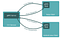# Platform independence

`\$env GOOS=windows GOARCH=amd64 go build`

# Testing and benchmarking built-in

`\$ go test -v -cover ./…`
`go test -bench=.`

# Why gRPC?In gRPC a client application can directly call methods on a server application on a different machine as if it was a local object, making it easier for you to create distributed applications and services.
`message SumRequest {    int32 a = 1;    int32 b = 2;}`
`service CalculatorService{    rpc Sum(SumRequest) returns (SumResponse) {};}`
`protoc calculatorpb/calculator.proto --go_out=plugins=grpc:.`
`\$ go run calculator_server/server.go`
`func (*server) Sum(ctx context.Context, req                                                *calculatorpb.SumRequest)                                           (*calculatorpb.SumResponse, error) {   fmt.Printf("Sum function was invoked with %v\n", req)   total := req.GetA() + req.GetB()   res := &calculatorpb.SumResponse {       Result: total,   }   return res, nil}func main() {   lis, err := net.Listen("tcp", "0.0.0.0:50051")   .   .   .   s := grpc.NewServer(opts...)           calculatorpb.RegisterCalculatorServiceServer(s, &server{})}`
`func main() {   fmt.Println("Calculator Client")   cc, err := grpc.Dial("localhost:50051", grpc.WithInsecure())   .   .   .   c := calculatorpb.NewCalculatorServiceClient(cc)   doUnary(c)}func doUnary(c calculatorpb.CalculatorServiceClient) {   req := &calculatorpb.SumRequest{      A: 3,      B: 6,   }   res, err := c.Sum(context.Background(), req)   if err != nil {      log.Fatalf("error calling the Sum service %v", err)   }   log.Printf("Result from sum:%d", res.GetResult())}`